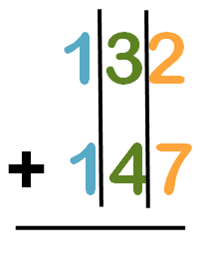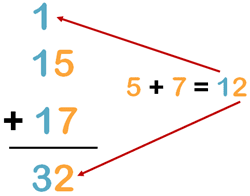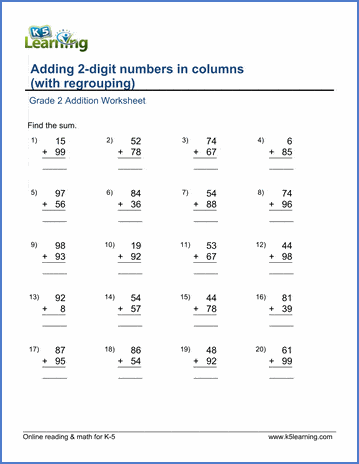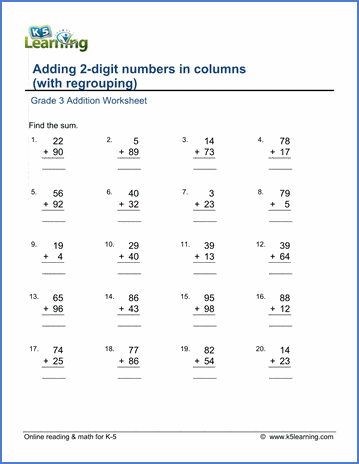# How to Solve Two-digit Addition with Regrouping

## What is regrouping?

To regroup means to re-arrange something into a new group or groups. So applied to addition, this would mean to regroup sums of numbers into a new group or groups.

Often regrouping in addition is more easily applied when you add numbers in columns, so that the ones are stacked above each other, the tens are stacked above each other, the hundreds are stacked above each other, etc.

The ones are in one group, the tens are in one group, the hundreds are in one group, etc.## When do you use regrouping?

Regrouping is used when the sum of the values in one place value column (or group as we referred to above) is more than nine.

For example, let’s add 15 + 17. In this case, you need to regroup. When you add the numbers in the ones place: 5 + 7, you have 12. When you break these units into their component parts, that is 2 ones and 1 ten.

The 1 belongs in the tens column, not the ones column, so you regroup it to the tens column.

When you add the numbers in the tens column you have the 1 + 1., and the ‘carried over’ 1 – so 1 + 1 + 1  = 3.

The answer is 15 + 17 = 32.## 2-digit addition with regrouping video

We’ve created a 60 second video to demonstrate visually how to add two 2-digit numbers that need regrouping: